# mathtutor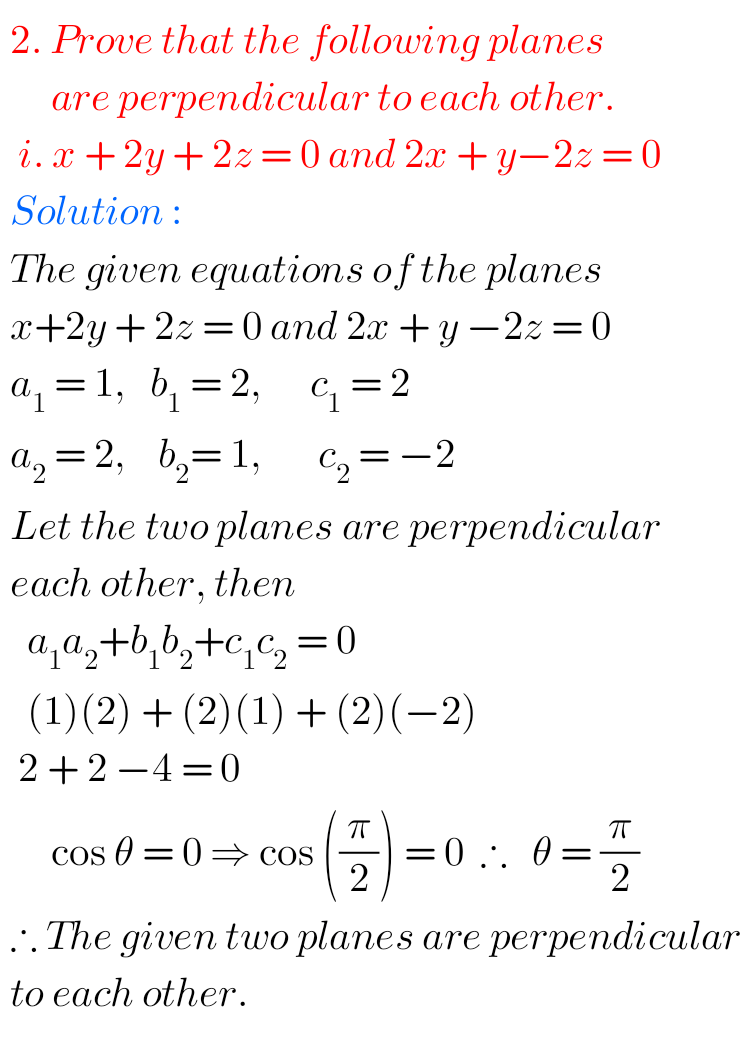## NIOS Maths class 12 solutions for Chapter 35 Plane Check your progress 35.2

Plane check your progress 35.2 solutions NIOS class 12 NIOS Mathematics 311 book 2 Module IX Chapter 36 Plane check your progress 35.2 solutions are given. You should study the textbook lesson Plane very well. You should also observe and practice all problems and solutions given in the textbook. Observe the given solutions and try …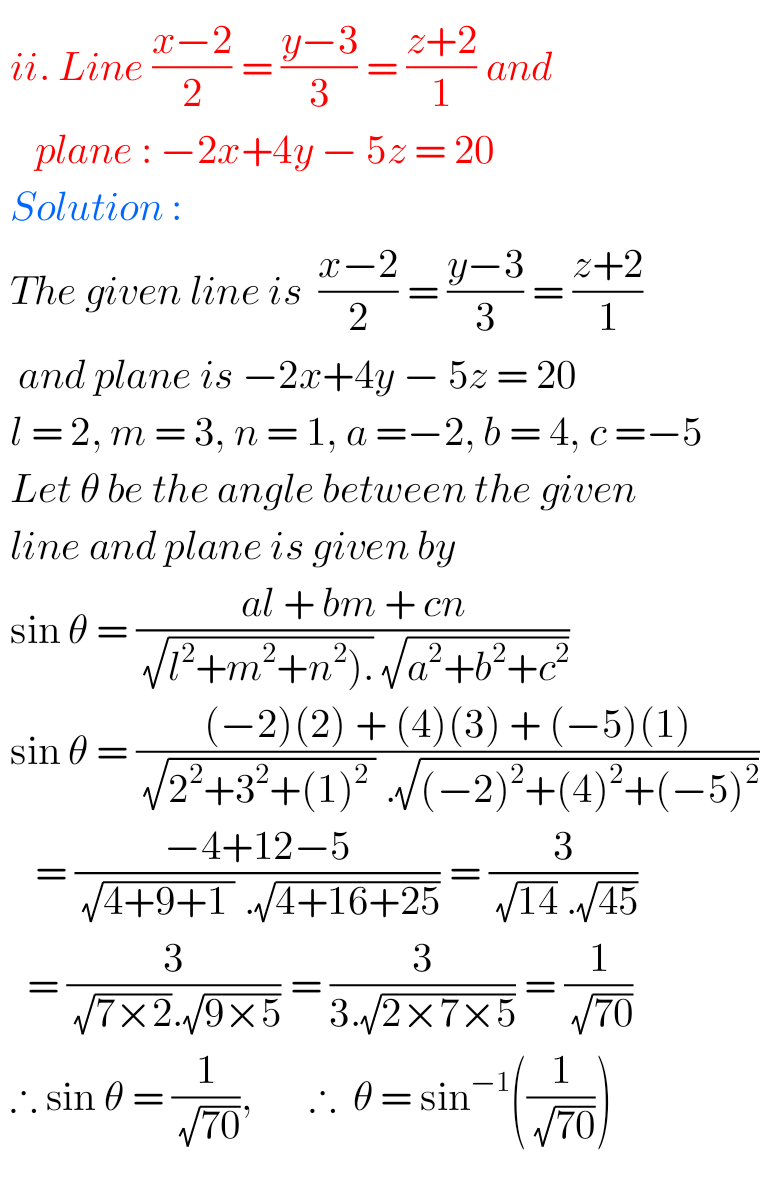## NIOS Maths Solutions for Class 12 chapter 36 Straight Line Check Your Progress 36.4

Straight line check your progress 36.4 solutions NIOS NIOS Mathematics 311 book 2 Module IX Chapter 36 check your progress 36.4 solutions are given. You should study the textbook lesson Straight Line very well. You should also observe and practice all problems and solutions given in the textbook. Observe the given solutions and try them …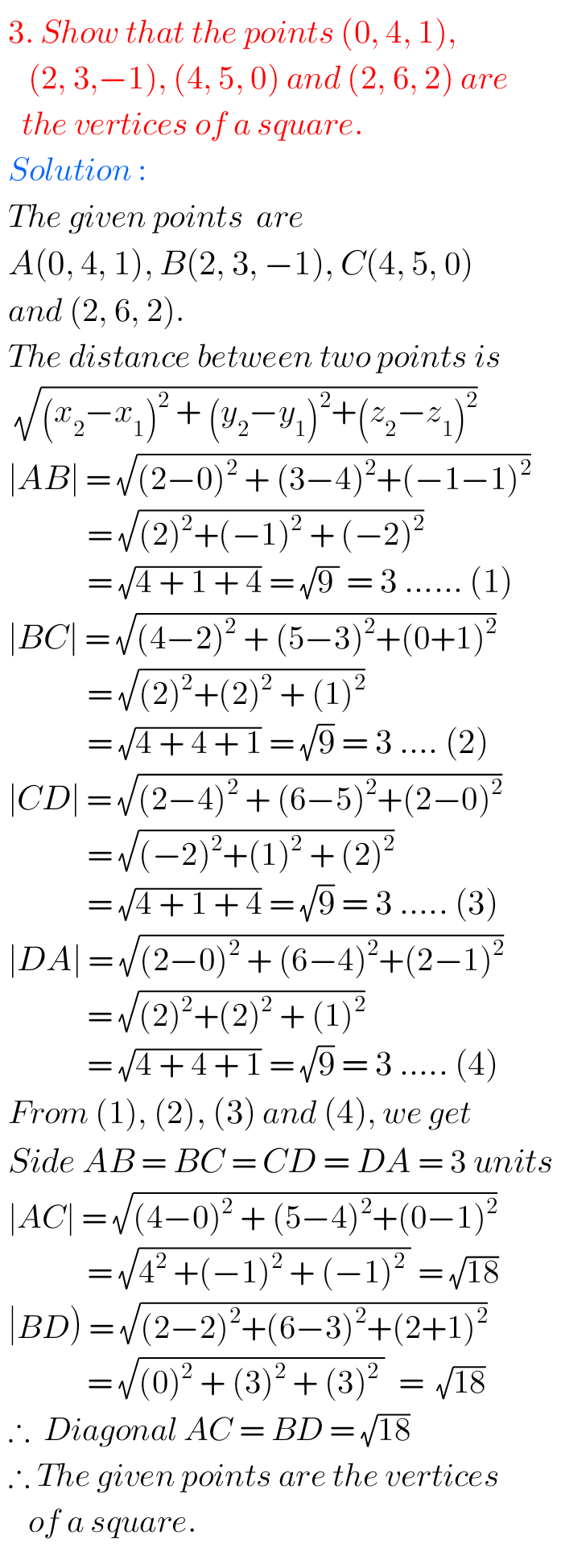## NIOS Mathematics class 12 book 2 chapter 33 Three Dimensional Geometry Terminal exercise solutions

Three Dimensional Geometry chapter 33 Terminal Exercise solutions NIOS NIOS Mathematics 311 book 2 Module IX Chapter 36 check your progress 36.2 solutions are given. You should study the textbook lesson Straight Line very well. You should also observe and practice all problems and solutions given in the textbook. Observe the given solutions and try …## NIOS Mathematics 311 book 2 solutions Senior secondary class 12

NIOS Senior Secondary Mathematics Book 2 solutions NIOS Senior secondary Mathematics 311 book 2 solutions are given for some chapters. You should study the textbook lessons very well. You should also observe and practice the example problems and solutions given in the textbook. Observe the given solutions and try them in your own method. M …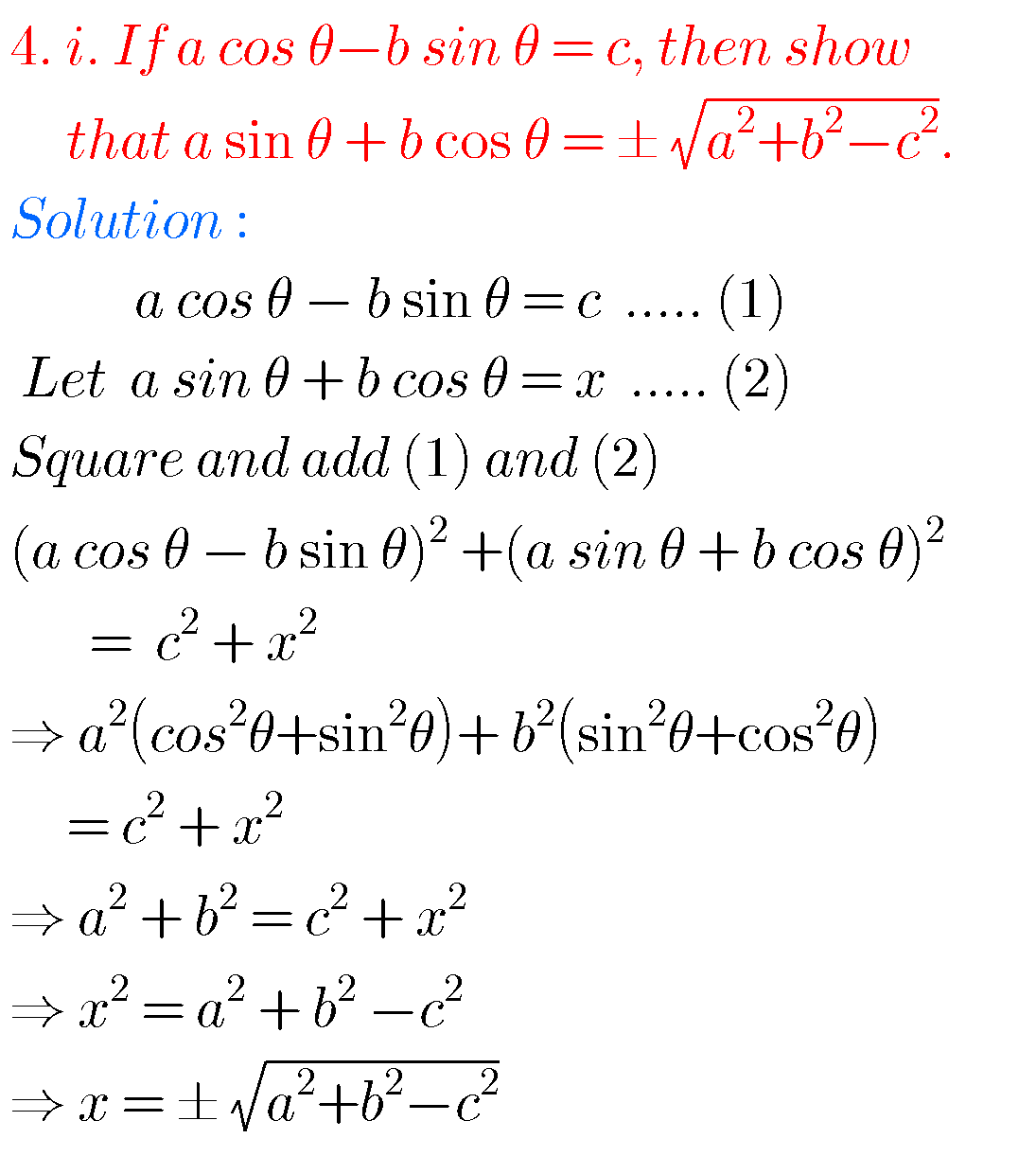## 1st Inter exercise 6(a) Solutions maths 1A ,chapter 6 Trigonometric Ratios upto Transformations

Inter maths 1A chapter 6 exercise 6(a) solutions Intermediate mathematics 1st year chapter 6 Trigonometric Ratios upto Transformations textbook solutions for exercise 6(a). These solutions are very easy to understand. Study the text boo lesson very well. Observe the example problems and solutions in the text book. Try them. Observe the given solutions and try …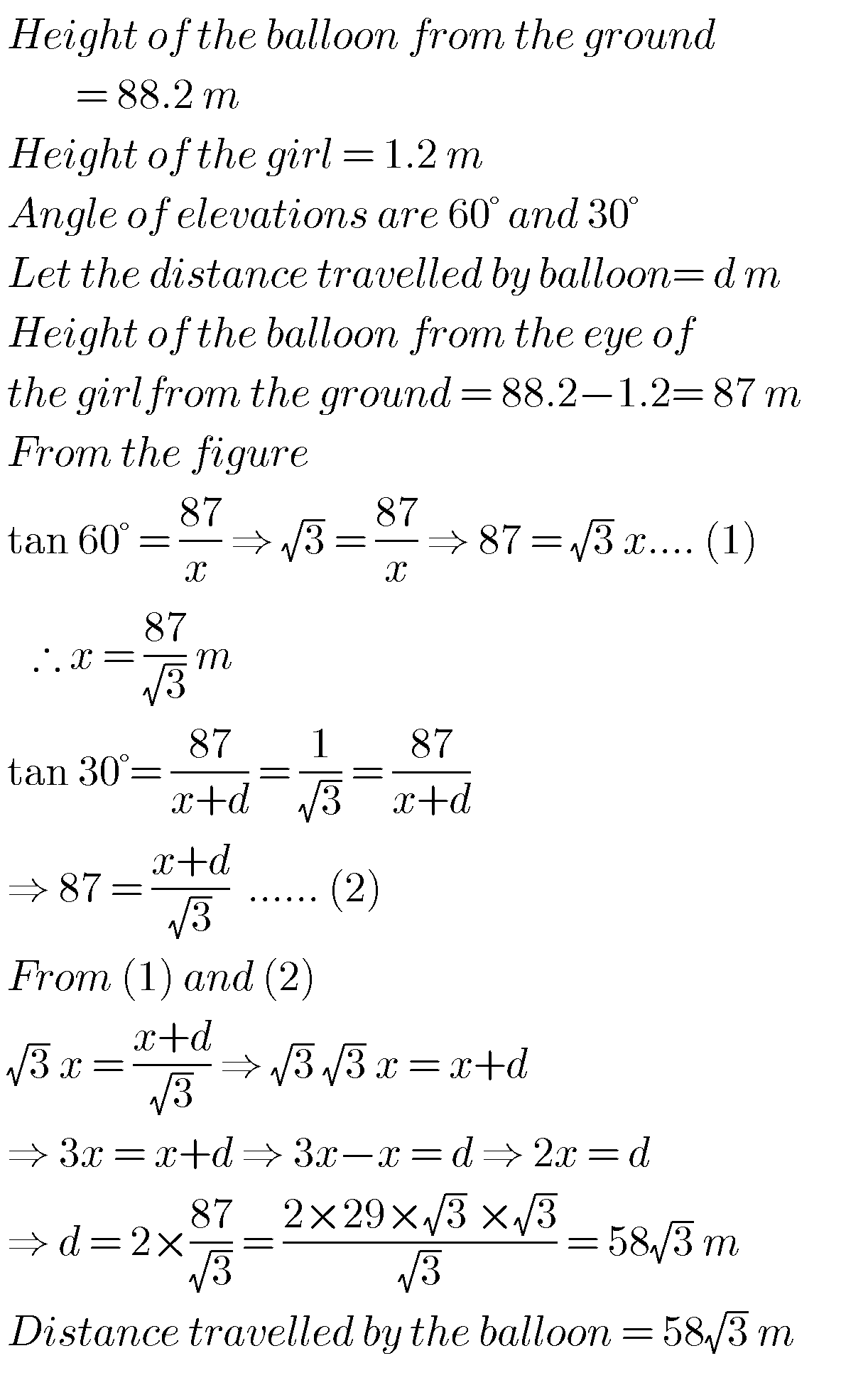## Maths solutions for Applications of Trigonometry class 10 ssc

Maths solutions for Applications of Trigonometry class 10 Exercise 12.1 and12.2 Tenth class mathematics chapter 12 exercises 12.1 and 12.2 solutions are given. These solutions are very easy to understand. First study the chapter 12 well for many times. Observe the example probems and solutions well. Try them in your own method. Next solve the …

## NIOS maths 311 solutions for Straight Line Chapter 36 check your progress 36.2

Straight line check your progress 36.2 solutions NIOS NIOS Mathematics 311 book 2 Module IX Chapter 36 check your progress 36.2 solutions are given. You should study the textbook lesson Straight Line very well. You should also observe and practice all problems and solutions given in the textbook. Observe the given solutions and try them …

## NIOS solutions for maths Three Dimensional Geometry Check your progress 33.3 solutions

NIOS maths 311 book 2 Three dimensional Geometry 33.3 solutions NIOS Mathematics 311 book 2 Module IX Chapter Three-Dimensional Geometry Check your progress 33.3 solutions are given. You should study the textbook lesson Three-Dimensional Geometry very well. You should also observe and practice all example problems and solutions given in the textbook. Observe the solutions …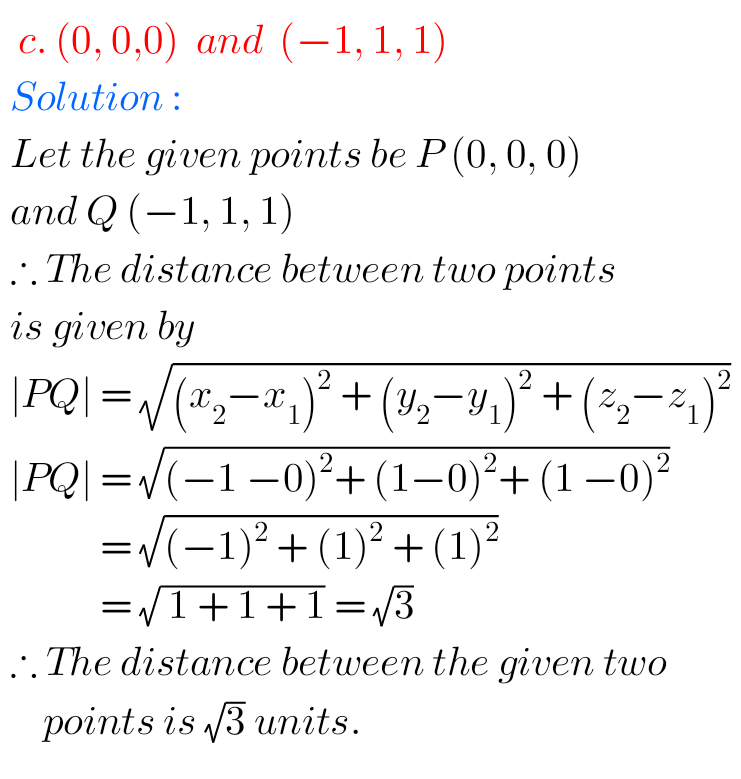## NIOS maths class 12 chapter 33 Three Dimensional Geometry Check your Progress 33.2 solutions

NIOS maths 311 book 2 Three dimensional Geometry 33.2 solutions NIOS Mathematics 311 book 2 Module IX Chapter Three-Dimensional Geometry Check your progress 33.2 solutions are given. You should study the textbook lesson Three-Dimensional Geometry very well. You should also observe and practice all example problems and solutions given in the textbook. Observe the solutions …## NIOS maths class 12 chapter 33 Three Dimensional Geometry check your progress 33.1 solutions

NIOS maths 311 book 2 Three dimensional Geometry 33.1 solutions NIOS Mathematics 311 book 2 Module IX Chapter Three-Dimensional Geometry Check your progress 33.1 solutions are given. You should study the textbook lesson Three-Dimensional Geometry very well. You should also observe and practice all example problems and solutions given in the textbook. Observe the solutions …